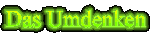Great Unifying Theory, Classical Physics, Theory Of Relativity & Quantum Theory

From physics at school anybody knows: W = m v² / 2 . Oddly enough the Theory Of Relativity says E = m c², this means twice the amount of energy for v = c and equal mass. Is it a contradiction? Usually this is "explained" by "relativistic" mass, speed or process. But nobody says, what it means, where the border between non-relativistic and relativistic speed is or what makes up a relativistic process.

Energy is energy, mass mass and speed speed (better space space and time time). Light speed is only the limiting speed of mass processes.

It can be explained by the Great Unifying Theory. To remember:

The more similar two things are, the stronger is the mutual influence of their behaviour.

Further explanation is given at Great Unifying Theory.

Two things means, that any change within a system is accompanied by an equal amount of change outside.

In classical physics this second change is not considered. So the amount of energy is apparently half of the real.

Einsteins formula describes the whole system and so the result is double to the classical physics model. It explains, what energy, mass, space and time are and how they are related. Relativity means, that it depends on the point of view, how a process is interpreted. The point can be that of energy, mass, space or time. The square of light speed in vacuum (299 792 km/s) is the factor, energy and mass are connected with.

For any process two interacting things are necessary and one of them is also the reference point. The change process occurs in equal amounts of positive or negative impulses: quantums. Their size depends on the properties of the interacting things (e.g. W = h f). They represent the elementary amounts of possible changes between two stable states of a property of a thing (object). Related to this property their possible values are 1, 0 and -1.

10.02.2003

Torsten Reichelt

Zur Hauptseite   Zur Textübersicht